Next: General Interaction Between Macrosystems Up: Statistical Thermodynamics Previous: Temperature

# Mechanical Interaction Between Macrosystems

Let us now examine a purely mechanical interaction between macrostates, where one or more of the external parameters is modified, but there is no exchange of heat energy. Consider, for the sake of simplicity, a situation where only one external parameter,, of the system is free to vary. In general, the number of microstates accessible to the system when the overall energy lies between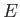and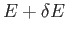depends on the particular value of, so we can write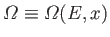.

Whenis changed by the amount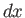, the energy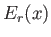of a given microstatechanges by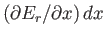. The number of states,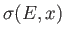, whose energy is changed from a value less thanto a value greater than, when the parameter changes fromto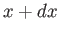, is given by the number of microstates per unit energy range multiplied by the average shift in energy of the microstates. Hence,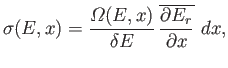(5.34)

where the mean value of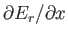is taken over all accessible microstates (i.e., all states for which the energy lies betweenand, and the external parameter takes the value). The previous equation can also be written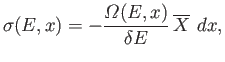(5.35)

where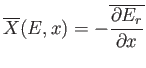(5.36)

is the mean generalized force conjugate to the external parameter. (See Section 4.4.)

Consider the total number of microstates whose energies lies betweenand. When the external parameter changes fromto, the number of states in this energy range changes by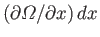. This change is due to the difference between the number of states that enter the range because their energy is changed from a value less thanto one greater than, and the number that leave because their energy is changed from a value less thanto one greater than. In symbols,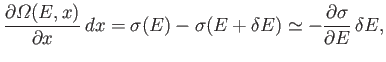(5.37)

which yields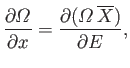(5.38)

where use has been made of Equation (5.35). Dividing both sides by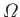gives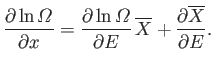(5.39)

However, according to the usual estimate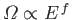(see Section 3.8), the first term on the right-hand side is of order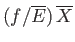, whereas the second term is only of order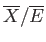. Clearly, for a macroscopic system with many degrees of freedom, the second term is utterly negligible, so we have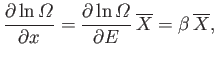(5.40)

where use has been made of Equation (5.30).

When there are several external parameters,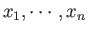, so that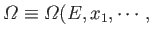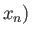, the previous derivation is valid for each parameter taken in isolation. Thus,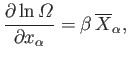(5.41)

where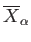is the mean generalized force conjugate to the parameter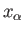. (See Section B.2.)Next: General Interaction Between Macrosystems Up: Statistical Thermodynamics Previous: Temperature
Richard Fitzpatrick 2016-01-25